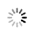# python获取当前系统时间

5月前 ⋅ 526 阅读

### ChatGPT的回答仅作参考：

```可以使用Python内置的datetime模块来获取当前系统时间。具体代码如下：

```python
import datetime

now = datetime.datetime.now()
print("当前时间为：", now)
```

```

```

```python
year = now.year
month = now.month
day = now.day
hour = now.hour
minute = now.minute
second = now.second
microsecond = now.microsecond

print("当前时间为：{}年{}月{}日{}时{}分{}秒{}微秒".format(year, month, day, hour, minute, second, microsecond))
```

```

``````

### 相关推荐